﻿ 20-sim webhelp > Toolboxes > Time Domain Toolbox > Optimization Methods

# Optimization Methods

In the Multiple Run Wizard you have to specify a result which will be optimized. The result is a function of model variables and the simulation run:

result = f(i,v1,v2,...)

with i the number of the simulation run. You also have to specify the parameters that should be varied to find the optimum result. We can group these parameters in a parameter vector:

parameter vector = p(i)

with again i the number of the simulation run. All methods in 20-sim for finding an optimum of the result use the same iterative process:

 1 The initial parameter vector is determined, e.g. p(1) and the corresponding function value f(1).
 2 A search direction r(1) and a stepsize s(1) are determined.
 3 Perform a new simulation run to find the parameter vector p(2) = p(1) + s(1)*r(1).
 4 Calculate f(2).
 5 When the f(2) is smaller than f(1) and the difference between the two is smaller than a given tolerance, stop the process. The optimum has been found.
 6 When the f(2) is smaller than f(1) and the difference between the two is larger than a given tolerance, proceed the process at step 2
 7 Otherwise a new stepsize and/or a new search direction are determined and the process is proceeded at step 3.

The choice of the stepsize is of importance for the speed an accuracy of the search process. A small stepsize will make the optimization process last very long. A large stepsize will make it less accurate. Most methods will therefore use a variable stepsize.

Of equal importance is the proper choice of the search direction. Methods for finding the search direction, can be divided in two groups: direct search methods and gradient search methods. The gradient of a function, is its slope at a certain point. Gradient search methods use this slope to find the optimal direction of search.

## Methods

The optimization methods that are supported in 20-sim will now be explained. The pictures at the right visualize the methods with two varying parameters x (horizontal) and y (horizontal) and the corresponding result (vertical).

### 1. Perpendicular Search (direct search)

The perpendicular search method uses a search direction that is always perpendicular to the parameter axis. This means that only one parameter at a time is varied. All other parameters keep the same value. After one step, a next parameter is taken and the process continues.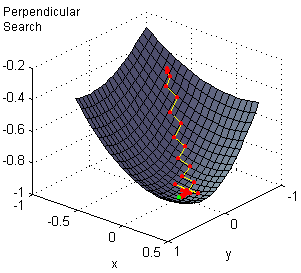### 2. Line Climber (direct search)

The line climber method uses a search direction that is always perpendicular to the parameter axis. This means that only one parameter at a time is varied. All other parameters keep the same value. After a minimum has been found the next parameter is varied and the process continues.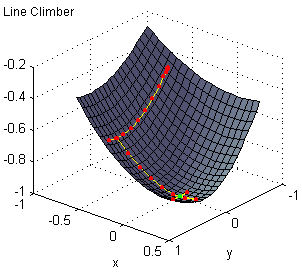### 3. Steepest Descent (gradient search)

The steepest descent method starts its search in the direction of the steepest slope. This direction is kept for each new step until a minimum has been found. Then a new search direction is determined and the process continues.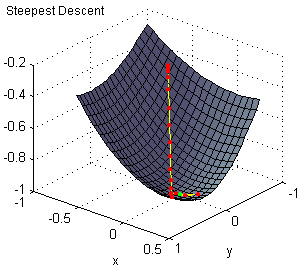### 4. Continuous Descent (gradient search)

The continuous descent method starts its search in the direction of the steepest slope. After each new step a new search direction is determined and the process continues.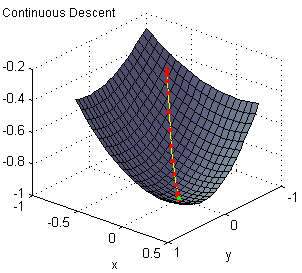### 5. Newton Raphson (gradient search)

The Newton Raphson method not only uses the gradient of a function, but also the second order gradient to determine the search direction. This direction is kept for each new step until a minimum has been found. Then a new search direction is determined and the process continues. Note: The method only converges for a positive second order gradient, i.e. near the minimum. This is shown in the figure to the right. For x = -0.7 and y = -0.9 the method does not converge. For x = -0.5 and y = -0.3 the method does converge.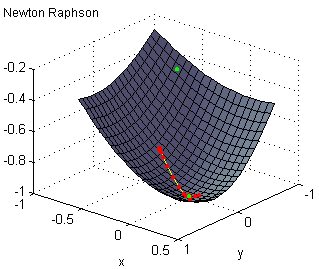### 6. Polack Ribiere (gradient search)

The Polack Ribiere method not only uses the gradient of a function, but also the second order gradient to determine the search direction. The second order gradient is estimated based on previous search directions. The search direction is kept for each new step until a minimum has been found. Then a new search direction is determined and the process continues.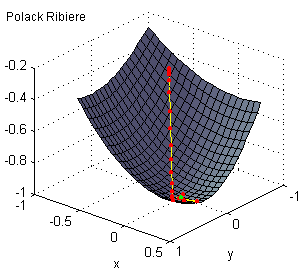### 7. Davidson Fletcher Powel (gradient search)

The Davidson Fletcher Powel method not only uses the gradient of a function, but also the second order gradient to determine the search direction. The second order gradient is estimated based on previous search directions. The search direction is kept for each new step until a minimum has been found. Then a new search direction is determined and the process continues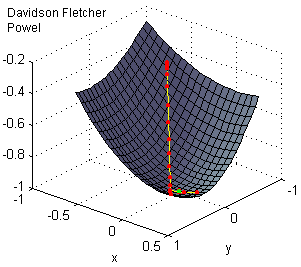### 8. Broydon Fletcher Goldfarb Shanno (gradient search)

The Broydon Fletcher Goldfarb Shanno method not only uses the gradient of a function, but also the second order gradient to determine the search direction. The second order gradient is estimated based on previous search directions. The search direction is kept for each new step until a minimum has been found. Then a new search direction is determined and the process continues.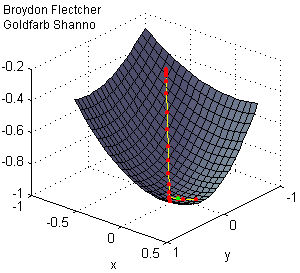## What method should be used?

There is no general answer to this question. Some remarks can however be made:

 1 Gradient search methods (3 to 8), need more steps (note that each step means a simulation run) to determine the gradient. The Newton Raphson methods needs the most steps, because this method also needs additional steps for the determination of the second order gradient.
 2 When the direction of the slope is not exactly the same as the search direction, direct search methods (1 and 2) may start to bounce, i.e. continuously change direction while making little progress. this is shown in the figure below: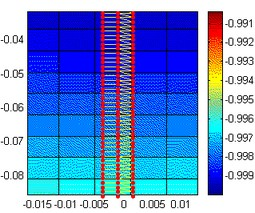Users are therefore advised to use methods 1 and 2 only for optimizations with one parameter, and use the methods 7 and 8 for optimizations with more parameters. Use the other methods for checking the results or educational purposes.